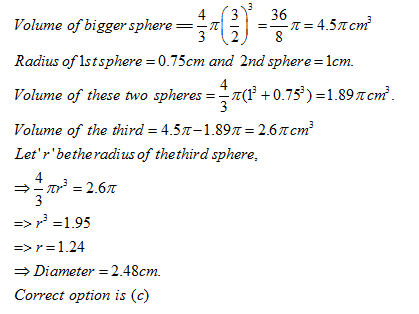• This is an assessment test.
• These tests focus on geometry and mensuration and are meant to indicate your preparation level for the subject.
• Kindly take the tests in this series with a pre-defined schedule.

## Geometry and Mensuration: Test 6

Congratulations - you have completed Geometry and Mensuration: Test 6. You scored %%SCORE%% out of %%TOTAL%%. You correct answer percentage: %%PERCENTAGE%% . Your performance has been rated as %%RATING%%
 Question 1
The total area of a circle and a square is equal to 5450 sq.cm. The diameter of the circle is 70 cms. What is the sum of the circumference of the circle and the perimeter of the square?
 A 360 cm B 380 cm C 270 cm D Cannot be determined
Question 1 Explanation:
Radius of circle = 70/2 = 35 cm.
Area of circle = ∏r2 x 35 x 35 = 3850 m2
Area of square = 5450 – 3850 = 1600 sq. m.
length of the square = 40 m.
Required sum =2π r + 4x40 = 2 x 22/7 x 35 + 160 = 220+160 = 380 cm
Correct option is (b)
 Question 2
Length of a rectangular blackboard is 8 m more than that of its breadth. If its length is increased by 7 m and its breadth is decreased by 4 m, its area remains unchanged. The length and breadth of the rectangular blackboard is
 A 24 m, 16 m B 20 m, 24 m C 28 m, 20 m D 28 m, 16 m
Question 2 Explanation:
Let the breadth of the board is 'x' m and it s length is x + 8 m
Its area is x(x + 8).
The new length is x + 15 and new breadth is (x - 4) m.
We have
x(x + 8) = (x + 15) (x - 4)
=> x2 + 8x = x2 + 11x - 60
=> 3x = 60
=> x = 20.
Hence the length and breadth of the board are 28m and 20 m respectively.
The correct solution is (c)
 Question 3
The area of a square is 1024 sq. cm. What is the respective ratio between the length and the breadth of a rectangle whose length is twice the side of the square and breadth is 12cm less than the side of the square?
 A 5: 18 B 16: 7 C 14: 5 D None
Question 3 Explanation:
Length of the square= √1024 =32cm
Length of the rectangle = 2x32 = 64 cm
and breadth = 32-12 = 20 cm.
Required ratio of length and breadth = 32: 20 = 8:5.
 Question 4
A cylindrical tub of radius 12 cm contains water up to a depth of 20 cm. A spherical iron ball is dropped into the tub and thus the level of water is raised by 6.75 cm. The radius of the ball is
 A 7.25 cm B 6 cm C 4.5 cm D 9 cm
Question 4 Explanation:
Let the radius of the ball is 'r'. We have
π ×122× (6.75) = 4/3 × π × r3
Solving we will get r= 9 cm.
 Question 5
A spherical ball of lead, 3 cm in diameter is melted and recast into three spherical balls. The diameter of two of these is 1.5 cm and 2 cm respectively. The diameter of the third ball is
 A 3 cm B 2.66 cm C 2.5 cm D 3.5 cm
Question 5 Explanation:Once you are finished, click the button below. Any items you have not completed will be marked incorrect.
There are 5 questions to complete.
 ← List →
 1 2 3 4 5 End

### Want to explore more Arithmetic Tests?

Get Posts Like This Sent to your Email
Updates for Free Live sessions and offers are sent on mail. Don't worry: we do not send too many emails..:)
Get Posts Like This Sent to your Email
Updates for Free Live sessions and offers are sent on mail. Don't worry: we do not send too many emails..:)